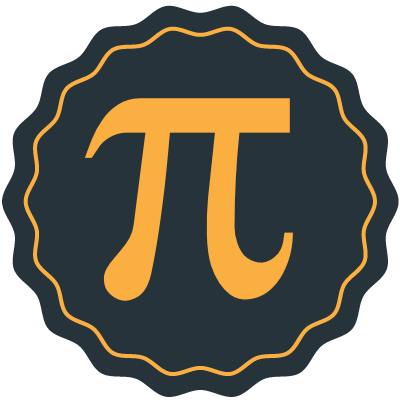### Please enable JavaScript to use CodeHSPlaylist: Hour of Code: Pi Day

By David B. — 10 activities
Have you ever wondered how many digits of Pi are really needed? Using Java loops and conditional statements, students will explore the impact of using more digits of Pi when calculating the orbit of Nasa Satellites. Note: Students should be familiar with basic loops, conditional, and print statements in Java before attempting this Hour of Code.
Video How Many Digits of Pi Does Nasa Use?
Example Using Math.PI
Exercise Calculate the Best Circumference
Video Using Math.Round
Example Rounding Pi
Exercise Circumference with 5 Digits of Pi
Exercise Loop through all decimal places
Exercise Customize the Output